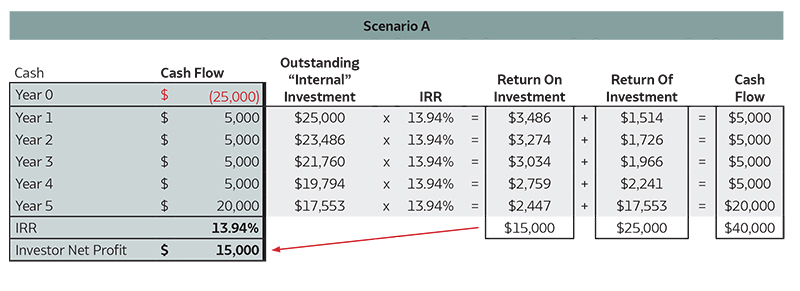# Why IRR Matters in Real Estate: Evaluating what is a good projected IRR

Feb 24, 2017

Investors use several metrics to evaluate real estate deals, including the internal rate of return (IRR), equity multiple, and cash on cash return. Of these, IRR is the most common and well-known metric utilized across real estate investments and asset classes.

## What Is a Target IRR?

Internal Rate of Return (IRR) is the average annual return over the lifetime of an investment. Sponsors and Investors use a complex formula to calculate the projected, or target, IRR for a deal – to project the returns they will achieve on a specific real estate project. This article will explain the formula used to calculate the target IRR, and how investors can use that target IRR to evaluate investment opportunities. To understand more about how the IRR translates into profits for investors, visit ArborCrowd’s Real Estate Investment Returns Calculator.

## How to Calculate the Target IRR in Real Estate Investing?

IRR is closely tied to another investment metric, the Net Present Value (NPV), which is essentially the difference between an investment’s market value and its total cost1. To understand IRR, we must first understand NPV.

## Net Present Value: Why it Is Used to Calculate IRR?

IRR is closely tied to another investment metric, the Net Present Value (NPV). Net present value (NPV) is the difference between the present values of cash coming in and going out over a period of time. Any investment with a positive NPV (a return greater than the cost of capital) will be profitable, and therefore, sponsors will always present deal offerings with positive projected NPVs.

Building on our understanding of NPV, let’s look at how the IRR is calculated.

IRR = The interest rate that makes the NPV equal to zero

The IRR is the discount rate that makes the net present value of all future cash flows and the initial total cost equal. To determine IRR, we can take the NPV formula below, define NPV as zero and solve for “r.”

$N\kern -1ptPV = -C_0 + \sum_{i=1}^T {C_i \over (1+r)^i}$

## IRR Real Estate Example

Microsoft Excel has a built-in function that does the IRR calculation for us, based on cash distributions over a projected period of time. Below is an illustration of how IRR works for a $25,000 investment in a deal with a projected hold period of roughly 5 years.The table on the left gives a quick overview of the cash flow over the five years, which for this particular scenario equates to an IRR of 13.94%. The right side shows what is going on behind the scenes for each year in terms of the Return on Investment and the Return of Investment. An investor would earn$15,000 in net profits over five years in Scenario A.

## What Is a Good IRR?

While it is helpful to understand how to calculate IRR, many investors still wonder what is a good IRR.

It is important to re-iterate that there are many factors at play when determining a good IRR. An investor first must decide how long they are comfortable with their capital outstanding and how much profit they project they will make at the end of that time period (the hold period of an investment).

Because an IRR is calculated based on time, it is possible for a shorter hold period to yield a higher IRR but with a lower equity multiple (less total dollars returned), while a longer hold period could yield a lower IRR but with a higher equity multiple (more total dollars returned).

Some investors might prefer a shorter hold period with a lower equity multiple but higher IRR. Other investors might be okay with a longer hold period with a greater equity multiple but lower IRR. This is entirely dependent on the investment objectives and preferences of the individual investor.

For a more in-depth analysis of how IRR works in different scenarios, read our article that explains what investors often look for when evaluating different IRRs across different types of investment opportunities.

### Key Takeaways:

• IRR is one of the key metrics investors use to evaluate real estate transactions.
• A good IRR is dependent on many factors and has to be evaluated in the context of an individual investor’s investment objectives and appetite for risk.
•